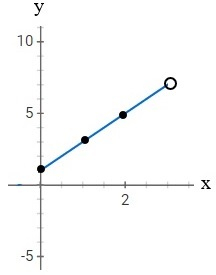# Divide line segment

Find the point P on line segment AB, such that |AP| = r |AB|. Coordinates of endpoints: A = (−2, 0, 1), B = (10, 8, 5), ratio r = 1/4.

x =  7
y =  6
z =  4

### Step-by-step explanation:Did you find an error or inaccuracy? Feel free to write us. Thank you!

Tips for related online calculators
Looking for help with calculating arithmetic mean?
The line slope calculator is helpful for basic calculations in analytic geometry. The coordinates of two points in the plane calculate slope, normal and parametric line equation(s), slope, directional angle, direction vector, the length of the segment, intersections of the coordinate axes, etc.
Looking for a statistical calculator?
Check out our ratio calculator.

#### Grade of the word problem:

We encourage you to watch this tutorial video on this math problem: